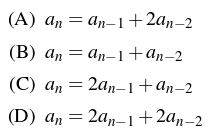# GATE | GATE-CS-2016 (Set 1) | Question 12

Let an be the number of n-bit strings that do NOT contain two consecutive 1s. Which one of the following is the recurrence relation for an(A) A
(B) B
(C) C
(D) D

Explanation: The least value of ‘n’ for the recursion would be 3.
For n = 1, number of strings = 2 (0, 1)
For n = 2, number of strings = 3 (00, 01, 10)
For n = 3, number of strings = 5 (000, 001, 010, 100, 101)
For n = 4, number of strings = 8 (0000, 0001, 0010, 0100, 1000, 0101, 1010, 1001)

This seems to follow Fibonacci series and the recurrence relation for it is an = an−1 + an−2.
Thus, B is the correct choice.

My Personal Notes arrow_drop_up

## Recommended Posts:

Article Tags :

Be the First to upvote.

Please write to us at contribute@geeksforgeeks.org to report any issue with the above content.